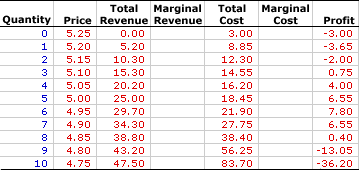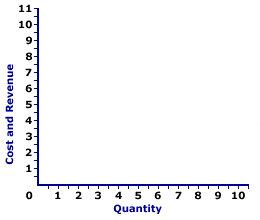Saturday  September 23, 2023
 AmosWEB means Economics with a Touch of Whimsy!DERIVATION, PRODUCTION POSSIBILITIES CURVE: A production possibilities curve, which illustrates the alternative combinations of two goods that an economy can produce with given resources and technology, is often derived from a production possibilities schedule. This derivation involves plotting each bundle from the production possibilities schedule as a point in a diagram measuring the two goods on the vertical and horizontal axes.MONOPOLISTIC COMPETITION, MARGINAL ANALYSIS:

A monopolistically competitive firm produces the profit-maximizing quantity of output that equates marginal revenue and marginal cost. This marginal approach is one of three methods that used to determine the profit-maximizing quantity of output. The other two methods involve the direct analysis of economic profit and a comparison of total revenue and total cost.
Monopolistic competition is a market structure with a large number of relatively small firms that sell similar but not identical products. Each firm is small relative to the overall size of the market such that it has some market control, but not much. In other words, it can sell a wide range of output at a narrow range of prices. This translates into a relatively elastic demand curve. If a monopolistically competitive firm wants to sell a larger quantity, then it must lower the price.

Comparable to any profit-maximizing firm, a monopolistically competitive firm produces the quantity of output in the short run that generates the maximum difference between total revenue and total cost, which is economic profit. This profit maximizing level of production is also achieved by the equality between marginal revenue and marginal cost. At this production level, the firm cannot increase profit by changing the level of production. The analysis of marginal revenue and marginal cost can be achieved through a table of numbers or with marginal revenue and marginal cost curves.

### Working the Numbers

A monopolistically competitive firm is presumed to produce the quantity of output that maximizes economic profit--the difference between total revenue and total cost. This decision can be analyzed using this table presented below. This table presents revenue and cost information for Manny Mustard's House of Sandwich, a hypothetical example of a monopolistically competitive firm, that results from the production and sale of Deluxe Club Sandwiches, a tasty luncheon consumed by residents of Shady Valley.

Because Manny produces a product with a large number of close but slightly different substitutes, he has some market control but not much. As such, he faces a negatively-sloped demand curve, but one that is relatively elastic. To sell a larger quantity, he must lower the price. Manny Mustard's status as a monopolistically competitive firm is reflected in this table.

The Sandwich Numbers• Quantity: The quantity of output produced by Manny Mustard, presented in the first column, ranges from 0 to 10 sandwiches. While, Manny Mustard could produce more than 10 sandwiches, this range is sufficient for the present analysis.

• Price: The second column presents the price received by Manny Mustard for selling sandwiches. As a price maker, the first and second columns represent the demand facing Manny for his sandwiches. The price Manny faces ranges from a high of \$5.25 per sandwich for a zero quantity to a low of \$4.75 per sandwich for 10 sandwiches. Manny Mustard can sell a larger quantity of sandwiches, but only by reducing the price. Manny Mustard is a price maker.

• Total Revenue: Total revenue is presented in the third column. This indicates the revenue Manny Mustard receives at each level of sandwich production. It is derived as the quantity in the first column multiplied by the price in the second column. Total revenue ranges from \$0 if no output is sold to a high of \$47.50 for selling 10 sandwiches. For example, selling 5 sandwiches generates \$25 of revenue and selling 8 sandwiches leads to \$38.80 of revenue.

• Total Cost: The fifth column presents the total cost incurred by Manny in the production of his sandwiches, ranging from a low of \$3 for zero output (which is fixed cost) to a high of \$83.70 for 10 sandwiches. Total cost continues to rise beyond 10 sandwiches, but this information is not needed for the present analysis. Producing 1 sandwich incurs a total cost of \$8.85. Producing 2 sandwiches incurs a total cost of \$12.30. Total cost rises as Manny produces more.

• Profit: The seventh column at the far right of the table contains economic profit, the difference between total revenue in the second column and total cost in the third column. It starts at -\$3, falls a bit to -\$3.85, rises to \$7.80, then falls to -\$36.20.
The task is to determine which sandwich production level provides the maximum profit using marginal revenue and marginal cost. The process goes something like this:
1. Marginal revenue indicates how much total revenue changes by producing one more or one less unit of output.
2. Marginal cost indicates how much total cost changes by producing one more or one less unit of output.
3. Profit increases if marginal revenue is greater than marginal cost and profit decreases if marginal revenue is less than marginal cost.
4. Profit neither increases nor decreases if marginal revenue is equal to marginal cost.
5. As such, the production level that equates marginal revenue and marginal cost is profit maximization.
The fourth and sixth columns are reserved for these two marginal measures. To display the numbers, click the [Marginals] button.
• Marginal Revenue: The fourth column displays marginal revenue, which declines from a high of \$5.20 for the first sandwich to a low of \$4.30 for the tenth sandwich. Because Manny is a monopolistically competitive firm with a small degree of market control, marginal revenue is less than price.

• Marginal Cost: The sixth column presents the marginal cost that Manny incurs in the short run for the production of sandwiches. It starts at \$5.85, declines to a low of \$1.65, then rises to \$27.45. The declining values are the result of increasing marginal returns and the rising values are due to decreasing marginal returns and the law of diminishing marginal returns.
How can these marginals be used to identify the profit-maximizing output level?
• First, a quick look at the profit column indicates that the profit-maximizing production is 6 sandwiches, which generates a profit of \$7.80. Click the [Profit Max] button to highlight this result.

• Second, with this outcome highlighted, note the corresponding marginal revenue and marginal cost values. Marginal revenue is \$4.70 for the sixth sandwich and marginal cost is \$3.45.

Manny increases production from 5 to 6 sandwiches because doing so generates \$4.70 of extra revenue and incurs only \$3.45 of extra cost, meaning profit increases by \$1.25 over the production of 5 sandwiches. Manny does not increase production from 6 to 7 sandwiches because doing so generates only \$4.60 of extra revenue but incurs \$5.85 of extra cost, meaning profit decreases by \$1.25.

As such, Feet-First Pharmaceutical settles in with the production of 6 sandwiches and achieves maximum profit. It cannot increase profit by changing production.

• Third, while marginal revenue and marginal cost might not appear to be equal for the profit-maximizing 6 sandwiches production (\$4.70 versus \$3.85), they really are. The reason is that the marginal numbers in the table actually represent discrete changes from one sandwich to the next. Reducing the size of the discrete change, say from 5.9999 sandwiches to 6 sandwiches, results in marginal revenue and marginal cost that are actually closer to \$4.65. At the limit of an infinitesimally small change, marginal revenue and marginal cost are exactly \$4.65.

While the equality between marginal revenue and marginal cost shows up better in a graph, the best practical method of identifying similar results, with a table of numbers, is to average the discrete changes on either side of the quantity. For example, the marginal cost AT the sixth sandwich of sandwich production is the average of the change from 5 to 6 (\$3.45) and from 6 to 7 (\$5.85), which is \$4.65. Marginal revenue AT the sixth sandwich is the average of the change from 5 to 6 (\$4.70) and from 6 to 7 (\$4.60), which is also \$4.65.

### Working the Curves

The Sandwich CurvesThe short-run production decision for a monopolistically competitive firm can be graphically illustrated using marginal revenue and marginal cost curves. The exhibit to the right is standing poised to display these curves.
• Average Revenue: First up is the average revenue curve, which can be seen with a click of the [Average Revenue] button. Because Manny Mustard is a monopolistically competitive firm, this average revenue curve is also its negatively-sloped demand curve.

• Marginal Revenue: A click of the [Marginal Revenue] button reveals the green line labeled MR that depicts the marginal revenue Manny receives from sandwich production. Because Manny is a price maker, this marginal revenue curve is also a negatively-sloped line, and it lies beneath the average revenue (and demand) curve. However, because Manny's demand is relatively elastic, these two curves are close together.

• Marginal Cost: A click of the [Marginal Cost] button reveals a red U-shaped curve labeled MC that represents the marginal cost Manny incurs in the production of sandwiches. The shape is based on increasing, then decreasing marginal returns.
The key for Manny is to identify the production level that gives the greatest level of economic profit. Profit is maximized with quantity of output found at the intersection of the marginal revenue and marginal cost curves, which is 6 sandwiches. Click the [Profit Max] button to highlight this production level.

To demonstrate why the equality between marginal revenue and marginal cost is the profit-maximizing production level, consider what results if marginal revenue is not equal to marginal cost:

• If marginal revenue is greater than marginal cost, as is the case for small quantities of output, then the firm can increase profit by increasing production. Extra production adds more to revenue than to cost, so profit increases.

• If marginal revenue is less than marginal cost, as is the case for large quantities of output, then the firm can increase profit by decreasing production. Reducing production reduces revenue less than it reduces cost, so profit increases.

• If marginal revenue is equal to marginal cost, then the firm cannot increase profit by producing more or less output. Profit is maximized.
Once the profit maximizing output is revealed, the last step is to identify the price charged by Manny. This is easily accomplished by clicking the [Price] button. Price is found by extending the 6-sandwich quantity upward to the average revenue curve, which is the demand curve. Buyers are willing to pay \$4.95 per sandwich if 6 sandwiches are sold.

Note that this \$4.95 price is greater than the \$4.65 marginal cost, which indicates that monopolistic competition does not technically achieve the price-equals-marginal-cost condition for efficiency. However, because the demand curve is relatively elastic, the difference is small.

 <= MONOPOLISTIC COMPETITION, LOSS MINIMIZATION MONOPOLISTIC COMPETITION, PROFIT ANALYSIS =>Recommended Citation:

MONOPOLISTIC COMPETITION, MARGINAL ANALYSIS, AmosWEB Encyclonomic WEB*pedia, http://www.AmosWEB.com, AmosWEB LLC, 2000-2023. [Accessed: September 23, 2023].

Check Out These Related Terms...

Or For A Little Background...

And For Further Study...
Search Again?RED AGGRESSERINE[What's This?] Today, you are likely to spend a great deal of time watching the shopping channel looking to buy either shoe laces for your snow boots or a rim for your spare tire. Be on the lookout for jovial bank tellers.Your Complete ScopeNorth Carolina supplied all the domestic gold coined for currency by the U.S. Mint in Philadelphia until 1828."People of mediocre ability sometimes achieve outstanding success because they don't know when to quit. "-- George Allen, U.S. senatorICAPMIntertemporal Capital Asset Pricing ModelA PEDestrian's Guide Xtra CreditTell us what you think about AmosWEB. Like what you see? Have suggestions for improvements? Let us know. Click the User Feedback link.| | | | | | | | | | |
| | | |

Thanks for visiting AmosWEB